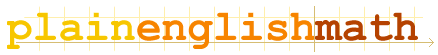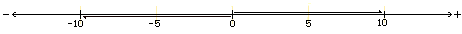You are here: MathematicsFundamentals → Numbers

# Numbers

Everyone knows what a number is! I'm not going to give a definition of number here (I wouldn't know where to begin). But I'd like to use this page to explain a few properties that numbers have; which I'll refer back to elsewhere on this site.
• Numbers are usually one-dimensional
• All numbers have a magnitude and a direction.

## One dimensional?

Allow me to provide a few examples: the number 2342.5 is one dimensional. The number 1 is one dimensional. The speed at which a car travels is one dimensional. In fact, just about all numbers you encounter in everyday life are one dimensional.

We live in a three dimensional world. You can move (1) left or right, while simultaneously moving (2) up or down and (3) foreward or backward. A sheet of paper is a two dimensional surface. A line drawn on the surface of a page can only run (1) left or right and (2) up or down at each point on that line. And a number like the total distance you walk each day, or the speed of a car, is one dimensional. You can only increase or decrease the distance you walk each day. The needle on a car speedometer can only move clockwise or counterclockwise. For instance, any number you add/subtract/multiply/divide the number 10 (or 1, or 2342.5, or ...) by, can only ever yield a result which is either larger or smaller than 10.

Imagine that the following number line is infinitely long. The number 10 can be expressed as a notch on the line, along with most other numbers. Adding a positive number to 10 yields a result which is situated to the right of 10. Subtracting a number from 10 (which is equivalent to adding a negative number to 10) gives a result shifted to the left of 10. You can't add two numbers together and get a result which is above or below the line itself! Being one dimensional, they are restricted to only existing along the length of the number line. (But you must be thinking; this is obvious! Well, in later sections, I'll discuss complex numbers and vectors; neither of which are restricted to a one dimensional number line - but you needn't worry about those at this stage.)## Magnitude?

The magnitude of a number is simply the distance of that number from zero. So the magnitude of the number 10 (written as |10| ) is 10, and |-10| (i.e. the magnitude of negative ten) is also 10; as both 10 and -10 are a distance of 10 units from zero.

## Direction?

The numbers 10 and -10 have equal magnitude, but if you take say, the number 5.3; and add the number 10 to it, you get a result situated 10 units to the right of 5.3 on the number line - that is to say, you get 5.3 + 10 = 15.3
If on the other hand, you add -10 to 5.3, you get a result shifted 10 units to the left of 5.3 on the number line.
That is, you get 5.3 + -10 = - 4.7

So in the case of these simple 1 dimensional numbers, each number can point in one of two directions: positive or negative. Just as the magnitude of a number is its distance from zero, the direction of a number is simply the direction in which you'd need to travel, starting from zero, in order to reach that number.

If you know the magnitude and direction of a number, then you know everything about that number.
Incidently, the zero point is often referred to as the origin, since the magnitudes and directions of all numbers are taken relative to zero.

## Scalars

Isn't the word scalar is a scary sounding term? Actually it's just the technical term for any one-dimensional number.
Scalars are so called because multiplying any number by a scalar simply scales its magnitude by that amount. Multiplying the number 10 by 2 for example, simply scales 10 up by a factor of 2, to give a result of 20. The direction of the number is unaffected, unless the number is multiplied by a negative scalar; in which case the direction is reversed. So while 2×10 = 20, -2×10 = -20. In the latter case, the direction is reversed due to scaling by a negative number.